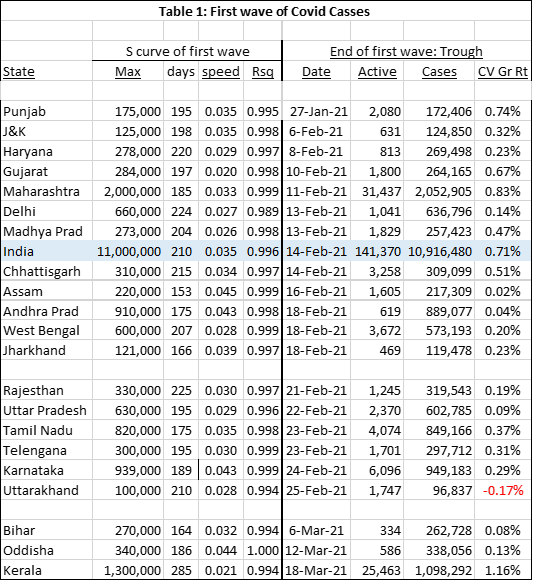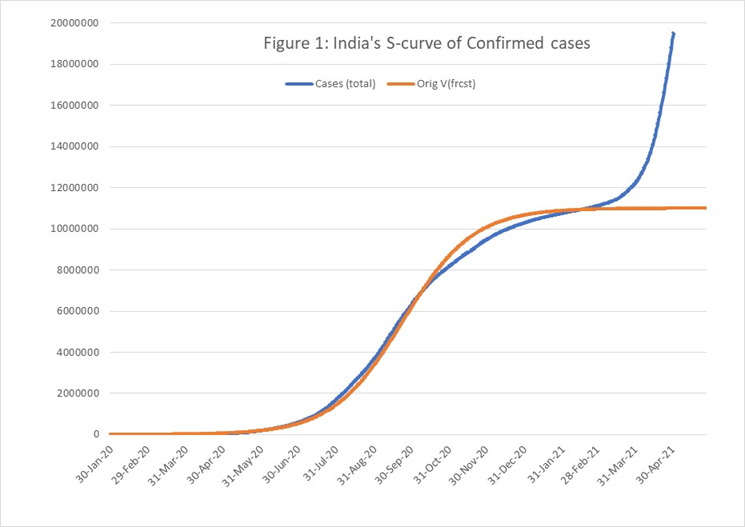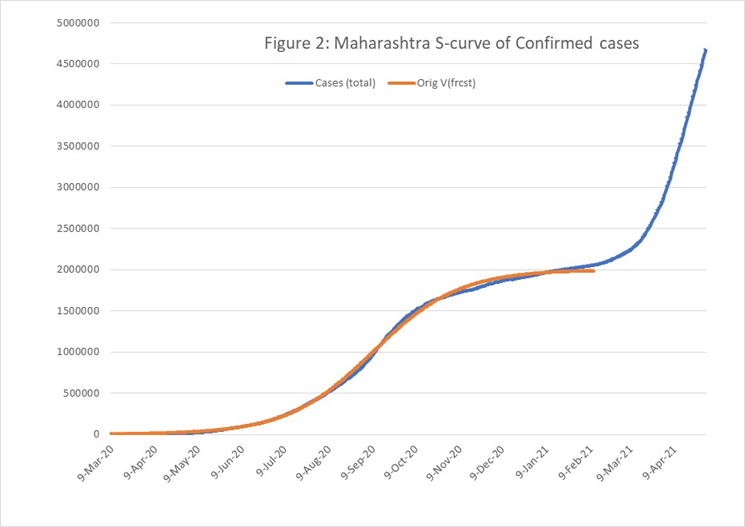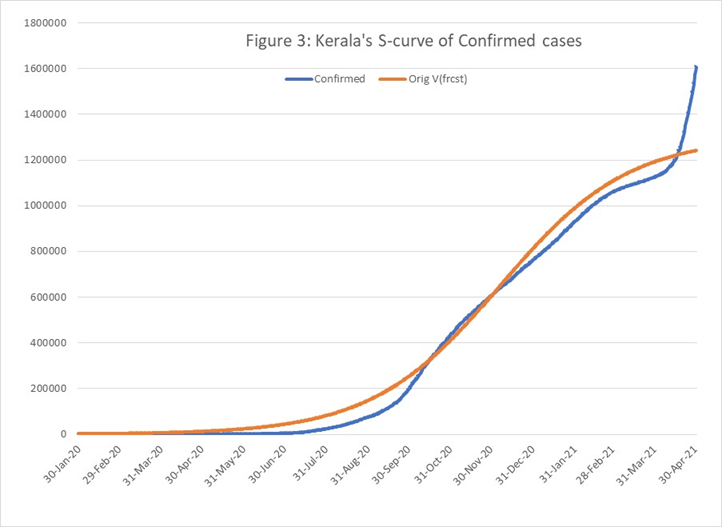# 1.    Introduction

In an earlier blog we had argued that India’s second wave was driven by virus mutations, not by much discussed events, like elections, farmers agitations and Religious functions like Kumbh mela, though these events undoubtedly contributed to the continuing spread of new mutations (29/4/21). In this note we present a State wise picture of the explosive spread of the new variants of the virus. In the next section we analyze the first wave and in section three the second wave. Section 4 puts the issue of public events in perspective, before concluding the paper.

# 2.    First Wave

We first analyze the first wave for the larger States, by identifying the point at which the rate of growth of new cases bottomed out. Then we fit the S curve, with 3 parameters, to the data, with best fit measured in terms of R squared. The results are presented in Table 1.Max is the upper limit of the S curve, days is the number of days it takes from start of cases (1st wave) to the inflection point at which the rate of daily (w-o-w) growth rate of case changes from acceleration to deceleration and speed is the slope parameter. Rsq  is R squared.

The row marked India (table 1), shows that it took 210 ten days to reach the inflection point and the R squared for the model fit is 0.996. It also shows the lowest growth rate of 0.71% was reached on 14th February 2021, with cumulative confirmed cases equal to 10.9 million(1.09cr) and the active cases equal to 1.4 lakhs. Punjab stands out as the earliest State to reach a trough in the rate of growth of cases, but there were 6 other States whose trough proceeded the All-India trough.  Bihar, Odisha and Kerala were the last three States to reach the trough, but Kerala stands out as the only State whose growth rate of cases was more than 1% even at the low point.

Figure 1 shows the actual trajectory of cases and the cases projected by the model. It’s seen that the second wave breaks through the projected trajectory of the first wave around mid-February 2021.All other States are found to follow a similar trajectory, with the second wave breaking out of the projected S curve trajectory, after the trough is reached. Figure 2 & 3 show the trajectory of Maharashtra’s covid cases, the fitted S-curve and the breakout of cases, above the forecast values, for Maharashtra and Kerala respectively. Maharashtra is a State where the second wave started early, around end -February 2021 and Kerala where it started late, around beginning of April 2021.Table 1 also shows the number of active cases and the total cases in each State on the data on which the lowest growth was reached. In Maharashtra, there were 31 thousand active cases and 2 million total cases on 11th February 2021 when the rate of growth was at its lowest point of 0.83%. Similarly there were 2800 active cases, and 1.7 lakh total cases when 27th January 2021.

--- part 2